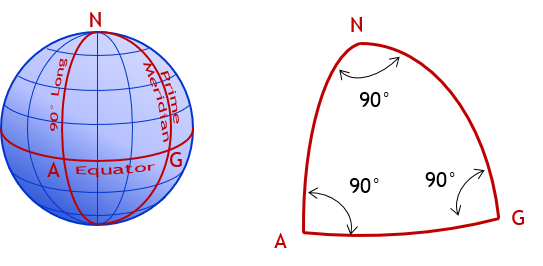## 1. Datum

A datum is a reference system from which measurements are made. When I state my height as (an optimistic) 5’11”, most will assume the distance is from where I’m standing to the top of my head - the floor is the datum. In surveying, we typically use either a flat two dimensional (plane) or three-dimensional (spheroid or ellipsoid) datum, Figure C-1, depending on the type of surveying.b. Two Dimensionalb. Three Dimensional Figure C-1 Data

While there are many different surveying activities and applications, traditionally all fall into one of two classifications based on the underlying reference system: Plane Surveying and Geodetic Surveying. While traditionally cut-and-dried, the distinction between these classifications has become very fuzzy due to rapidly evolving technology. Despite this, starting with the classifications give us a framework on which to build. As we look at various concepts we will indicate distinctions between classifications as necessary.

## 2. Plane Surveying

Plane surveying is based on a the earth’s surface being flat, a plane, Figure C-2.Figure C-2 Plane Surveying

Using a flat two dimensional reference surface simplifies many computations. Lines are straight and related to angles by plane trigonometry. The angle sum for any triangle is 180°00’00” regardless the combination of sides and angles, Figure C-3.Σ(angles) = 180°00’00” Figure C-3 Plane Triangle

Over small areas, plane surveying principles may be used without introducing significant errors.

Since the reference surface is flat, gravity directions (and hence vertical lines) are everywhere parallel. Horizontal lines are perpendicular to gravity along their entire lengths, Figure C-4.Figure C-4 Vertical Lines in Plane Surveying

## 3. Geodetic Surveying

The physical earth is three dimensional and quite irregular due to mass anomalies, rotational wobble, centrifugal force, lunar attraction, etc. Geodetic Surveying takes into account the size and shape of the earth, Figure C-5.Figure C-5 Geodetic Surveying

Complex mathematical models are used to create a three dimensional datum to approximate the earth and measurements must be transferred from the physical earth to and from the datum. Vertical lines converge making computations more complex. Unlike flat triangles in plane surveying, geodetic triangles are spherical with angle sums exceeding 180°, sometimes by quite a bit. For example, in a triangle created by intersecting the Prime Meridian, 90th meridian, and the Equator, Figure C-6, each angle is 90° for a total of 270°.Σ(angles) = 270°00’00” Figure C-6 Spherical Triangle

In Geodetic Surveying, because the earth is curved, gravity lines converge. A horizontal line is perpendicular to a vertical line at only a single point, Figure C-7.Figure C-7 Vertical Lines in Geodetic Surveying
Hits: 6919